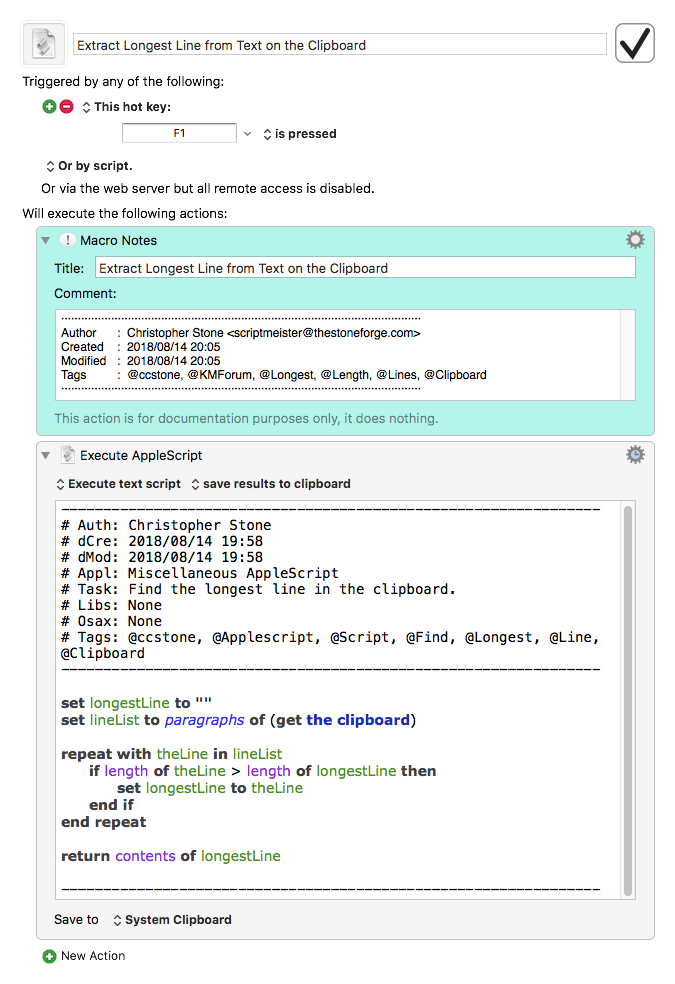# “Copy, sort by length and paste” Macro

In my line of work I often need to find the longest line in some text.
For example to test if a business card design can accommodate the names supplied.

For this I use the macro below.

Keyboard Maestro 8.2.4 “Copy, sort by length and paste” Macro

3 Likes

Nice shell code for sort lines based on lengthIs there an advantage to creating the temporary file in this macro over doing this:

i.e. taking input from the system clipboard.

1 Like

No advantage. I just did not know how to get the clipboard in without writing the file first.
Thanks for the input.

1 Like

5 posts were split to a new topic: TIP: How to Use Standard In (stdin) in Shell Scripts

And I forgot to mention.
I have not made the shell code myself. Not that cleverI found it here:

and if anyone wanted to include this kind of thing in an Applescript or Javascript context, we could paste a generic maximumBy function and then:

Applescript

``````maximumBy(comparing(|length|), ¬
paragraphs of (the clipboard))
``````

Javascript

``````maximumBy(
comparing(length),
lines(
)
);
``````

For example:
Longest line in clipboard (Applescript).kmmacros (20.1 KB)

Applescript source

``````on run

maximumBy(comparing(|length|), |lines|(the clipboard))

end run

-- GENERIC FUNCTIONS ------------------------------------------------

-- https://github.com/RobTrew/prelude-applescript

-- comparing :: (a -> b) -> (a -> a -> Ordering)
on comparing(f)
script
on |λ|(a, b)
tell mReturn(f)
set fa to |λ|(a)
set fb to |λ|(b)
if fa < fb then
-1
else if fa > fb then
1
else
0
end if
end tell
end |λ|
end script
end comparing

-- foldl :: (a -> b -> a) -> a -> [b] -> a
on foldl(f, startValue, xs)
tell mReturn(f)
set v to startValue
set lng to length of xs
repeat with i from 1 to lng
set v to |λ|(v, item i of xs, i, xs)
end repeat
return v
end tell
end foldl

-- length :: [a] -> Int
on |length|(xs)
length of xs
end |length|

-- lines :: String -> [String]
on |lines|(xs)
paragraphs of xs
end |lines|

-- max :: Ord a => a -> a -> a
on max(x, y)
if x > y then
x
else
y
end if
end max

-- maximumBy :: (a -> a -> Ordering) -> [a] -> a
on maximumBy(f, xs)
set cmp to mReturn(f)
script max
on |λ|(a, b)
if a is missing value or cmp's |λ|(a, b) < 0 then
b
else
a
end if
end |λ|
end script

foldl(max, missing value, xs)
end maximumBy

-- Lift 2nd class handler function into 1st class script wrapper
-- mReturn :: First-class m => (a -> b) -> m (a -> b)
on mReturn(f)
if class of f is script then
f
else
script
property |λ| : f
end script
end if
end mReturn
``````

Javascript source

``````(() => {

const main = () =>
maximumBy(
comparing(length),
lines(
)
);

// GENERIC FUNCTIONS ----------------------------------

// https://github.com/RobTrew/prelude-jxa

// comparing :: (a -> b) -> (a -> a -> Ordering)
const comparing = f =>
(x, y) => {
const
a = f(x),
b = f(y);
return a < b ? -1 : (a > b ? 1 : 0);
};

// length :: [a] -> Int
const length = xs => xs.length;

// lines :: String -> [String]
const lines = s => s.split(/[\r\n]/);

//  Ordering: (LT|EQ|GT):
//  GT: 1 (or other positive n)
//    EQ: 0
//  LT: -1 (or other negative n)

// maximumBy :: (a -> a -> Ordering) -> [a] -> a
const maximumBy = (f, xs) =>
0 < xs.length ? (
xs.slice(1)
.reduce((a, x) => 0 < f(x, a) ? x : a, xs)
) : undefined;

// JXA ------------------------------------------------

// standardSEAdditions :: () -> Application
Object.assign(Application('System Events'), {
});

// MAIN -----------------------------------------------
return main();
})();
``````
1 Like

And this one sort with the longest first in the list.
Actually more what I need.

1 Like

Hey Guys,

Here's one more for posterity.

I could have made it a bit faster, but I went for sheer simplicity.

It runs nearly instantly on 1,000 lines of text, and that's fast enough for me.

Copy and paste is left up to the user, so you may need to modify it a bit for your task.

-Chris

Extract Longest Line from Text on the Clipboard.kmmacros (5.1 KB)1 Like

A post was merged into an existing topic: Simplicity vs Complexity

A post was split to a new topic: Simplicity vs Complexity